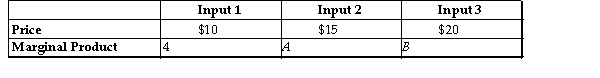[Solved] -Refer to the Table Above-Refer to the table above. If A = 6 and B = 12, which of the following is true?

A)The firm should buy more of Input 1 and less of Inputs 2 and 3.
B)The firm should buy more of Input 2 and less of Inputs 1 and 3.
C)The firm should buy more of Input 3 and less of Inputs 1 and 2.
D)The firm is minimizing costs.

10+ million students use Quizplus to study and prepare for their homework, quizzes and exams through 20m+ questions in 300k quizzes.

Explore our library and get Economics Homework Help with various study sets and a huge amount of quizzes and questions

Upload material to get free accessInvite a friend and get free accessSubscribe and get an instant access